# Unique Challenges of Managing Inductive Knowledge

David Jensen
Experimental Knowledge Systems Lab
Computer Science Department
University of Massachusetts
Amherst, MA 01003-4610
jensen@cs.umass.edu

### Abstract:

Tools for inducing knowledge from databases, often grouped under the term knowledge discovery, are becoming increasingly important to organizations in business, government, and science. However, relatively little attention has been paid to the long-term management of induced knowledge. Induced knowledge presents unique challenges, including managing statistical significance and inductive bias. These two challenges have important implications for valid and efficient knowledge management.

# Executive Summary

Algorithms for inducing knowledge are becoming increasingly important in business, government, and science. In the past three years, a large number of commercial systems for knowledge discovery have been developed and fielded, and these systems are being actively applied by hundreds of organizations. This increasing interest has also been reflected in the research community, where knowledge discovery and data mining are the subject of several new conferences, journals, and books.

Typically, these systems are concerned with producing knowledge. They analyze a data sample to produce a set of inductive inferences that are then applied directly by human users or encoded into other software. However, knowledge-based systems are increasingly coming into long-term use within organizations. This implies the need to explicitly maintain and manage all knowledge, including knowledge that is derived inductively.

This paper argues that induced knowledge has at least two unique characteristics, and that these characteristics impose special requirements on knowledge management systems. The first characteristic concerns statistical significance, characterized by a non-zero probability that any observed relationship may be due to random variation alone. The need to evaluate statistical significance implies that knowledge management systems must be at least loosely coupled with systems for two other functions: data management and induction. Knowledge cannot simply be induced and then permanently transferred to a knowledge management system. Instead, continued communication between these systems is necessary to effectively manage induced knowledge. The second unique characteristic of induced knowledge is inductive bias, the ordering of possible models imposed by a search procedure. Inductive bias provides additional reasons that knowledge management systems should be coupled with systems for induction.

If knowledge management systems contain inductively derived knowledge, but fail to account for these unique challenges, they will fall prey to several pathologies. These include faulty estimates of validity, missed opportunities to discover useful relationships, and redundant search efforts.

The remaining three sections support these claims. The first two sections introduce statistical significance and inductive bias, provide examples, and present implications. Readers who already understand these concepts may wish to skip the front portions of these sections, but they are provided for completeness. The third section discusses briefly system design issues in the context of these characteristics.

# Statistical Significance

A particular type of uncertainty is associated with all induced knowledge. There is a probability p that any observed relationship is merely due to random variation. Even if there is perfect correlation between two variables, there is still a non-zero probability that the relationship occurred by chance alone.

## An Example

For example, consider the simple data sample shown in Figure 1. The model M, here represented as a rule, expresses a relationship between two variables, and the data sample D provides a way of empirically evaluating the accuracy of that model. The relationship expressed by M in the data sample D can be compactly expressed by the contingency table in Figure 1.Figure 1: Example Significance Test

Assuming that M was derived independently of D, it is possible to estimate the probability p using two things: 1) a statistic, and 2) its reference distribution. A statistic summarizes the quality of a relationship in a single scalar measure. A standard statistic for the type of table in Figure 1 is the G statistic.whereis the number of occurrences, or frequency, in the cell i,j andis the expected value of that cell. In this case, the expected value is, whereis the total frequency in row i,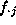is the total frequency in column j, andis the total of all cells in the table. The table in Figure 1 results in a G value of 3.55.

A reference distribution indicates the frequency of a statistic's values that would be expected under the null hypothesis -- in this case, the hypothesis that the variables V1 and V2 are independent. The reference distribution for G is a chi-square distribution with (r-1)(c-1) degrees of freedom, where r is the number of rows and c is the number of columns in the table. The table in Figure 1 has one degree of freedom.

As shown schematically in Figure 1, 5.9% of the reference distribution for G is equal or greater to 3.55, indicating that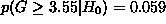, where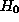is the null hypothesis. The probability p can be very small, but it is always non-zero.

## The Meaning of Statistical Significance

In general, statisticians refer either to p directly or to statistical significance. A relationship is statistically significant if its value of p is less than some preset threshold, typically 5% or 10%. An alternative approach with exactly the same effect is to determine whether a G value exceeds a certain critical value -- the value of G corresponding to. The 10% critical value for G is 2.706 -- the value above which 10% of G's distribution lies. The model in Figure 1 is significant at the 10% level because its G value exceeds the 10% critical value.

The probability p is distinct from what could be called the inferential uncertainty of a relationship, the uncertainty associated with making a particular inference. The model M might be said to have an inferential uncertainty of 20%; based on D there appears to be a 20% probability of making an incorrect inference when using the rule. Statistical significance and inferential uncertainty are related, but the relationship is mediated by several other factors discussed below.

Statistical significance is also distinct from the probability that a particular model is ``correct.'' It is a mistake to think that, merely because a model is statistically significant, that it is necessarily correct. Indeed, the actual relationship could have a different functional form than the induced model, less (or more) inferential uncertainty, different parameter values, additional (or fewer) variables, latent variables, and many other differences.

Instead of a guarantee, statistical significance is only a minimum indicator of validity. If an observed relationship can be explained entirely as chance variation (e.g., p is very large), then there is little need to investigate further. If p is very small, then additional questions about the form and content of the relationship are worth investigating.

The discussion above suggests a design requirement for knowledge management systems: an estimate of p should be calculated and stored along with knowledge that has been derived inductively. This estimate can be used, along with other information, to judge the validity of an induced model. Different uses may imply different desired levels of statistical significance. For example, medical treatments that are expensive or dangerous might be required to meet higher standards of statistical significance than treatments that are cheap and relatively benign.

## Why Statistical Significance Can Be Difficult to Determine

Based on the example above, calculating p seems relatively straightforward. Unfortunately, the example is misleading in at least two important respects -- M was evaluated on only a single sample of data and M was assumed to arise independently of that sample. In reality, knowledge management systems will have to relate rules such as M to more complex and evolving samples of data and such rules will be derived based on extensive search of those same samples.

These factors raise serious issues for knowledge management. The complexities arise because p, for a given model M, depends on both the data and the method used to find the model.

The dependence on data is reasonably obvious. The probability p depends on the strength of the relationship identified in the data and on the size of the sample available to test the relationship. For example, consider the three contingency tables in Figure 2. In each case, the associated p value was determined by comparing the value of the G statistic to its reference distribution. Both tables a and b have the same total frequency, but table b expresses a stronger relationship and has a correspondingly lower value of p. Similarly, tables b and c have a relationship of the same strength (in terms of inferential uncertainty), but table c has a vastly lower p value because it corresponds to a sample of larger total size.Figure 2: Three Contingency Tables

In addition to depending on the data, p depends on the number of models examined by an induction algorithm. Consider an induction algorithm that examines n models --. Under the null hypothesis, each model's G statistic has a 10% probability of exceeding 2.706, the 10% critical value for G. However, the probability that one of the models' G statistic exceeds 2.706 is almost certainly larger. If the predictions of each of the models are independent, then:whereis the probability that at least one of the n models' G values exceeds 2.706 andis the probability that a single model's G value exceeds 2.706. For example, ifis 0.10 and 20 models are examined, then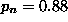. In practice, induction algorithms compare thousands or tens of thousands of different models by varying the functional form, variables used, or settings of parameters. As a result, adjusting for these multiple comparisons becomes essential to accurately estimate p.

Equation 2 is one of a class of Bonferroni equations, commonly used to adjust statistical tests for multiple comparisons and more recently applied to induction algorithms. The adjustment is necessary because the reference distribution for G is constructed under the assumption of a single comparison of a model to a data sample. Making multiple comparisons renders this reference distribution inaccurate.

A Bonferroni equation assumes that the comparisons are independent -- i.e., that the results of one comparison tell us nothing about the outcome of another comparison. Unfortunately, multiple comparisons by induction algorithms are rarely independent. Multiple models generated during search often have similar structure and use similar variables. As a result, the comparisons are not independent, potentially rendering the Bonferroni equation inaccurate. To a first approximation, however, potential correlation can sometimes be ignored, and we will not deal further with this issue here.

In addition to a Bonferroni equation, there are several other techniques that can be used to compensate for multiple comparisons even when those comparisons are non-independent. These include randomization tests, a method of empirically constructing reference distributions based on analyzing random data, and cross-validation, a method of systematically providing fresh data for evaluating the results of extensive search.

## Potential Pitfalls and How to Avoid Them

All of these techniques, however, require information about which data were used to construct the model and what alternative models were tested during the construction. To make this more concrete, consider the following situations:
• Unintentional data reuse: A model M is derived based on a sample of data D. It is stored without any references to data. Later, M is tested on data to verify its accuracy. Without records of how M was derived, it would appear that M has been independently verified. Unfortunately, M was ``verified'' on D, the same sample used to derive it. Potential mistakes of this kind can only be avoided if a link is maintained to the original data used to derive a model.
• Uncoordinated, distributed search: Twenty analysts work independently on data sample D, each evaluating the accuracy of a different model. One analyst's model is statistically significant at the 10% level. Without the information that other analysts are conducting similar analyses, it would appear that a significant relationship has been identified. Considering the actions of all the analysts (e.g., by using equation 2), the result is not statistically significant. This indicates the importance of maintaining records of the uses of different data samples.
• Ignoring sample size: Two models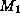andare induced and stored with estimates of their inferential uncertainty -- the percentage of incorrect predictions. Later, they are compared and found to be equally useful. Unfortunately, model's estimate was based on data samplewith 1000 instances; model's estimate was based on data samplewith only 10 instances. While the two models have identical inferential uncertainty, the first estimate is far more reliable. Judgments of this kind can only be made if a knowledge management system retains some information about statistical significance or the data sample used to derive a model.
• Incremental induction: A model is developed on a small data sample and, while suggestive of an interesting relationship, it does not exceed a prespecified critical value. Another small sample of data becomes available later, but it is also too small to confer statistical significance to the model. However, the relationship would be significant if considered in the context of both data samples together. This indicates the importance of maintaining both tentative models and links to the original data.
These examples indicate a few of the situations where statistical significance is both an important characteristic of induced knowledge to consider, and why it holds implications for the design of knowledge management systems. A second issue, inductive bias, also has important implications for the knowledge management.

# Inductive Bias

All induction algorithms search an explicit or implicit space of possible models. Because this space must be finite, the algorithms necessarily exclude some possible models from their search space. In addition, induction algorithms impose an ordering on the models within their search space. They select some models over others, based on apparent accuracy, relative complexity, and other factors.

Machine learning researchers label these factors inductive bias. Inductive bias is a necessary characteristic of any induction algorithm. Indeed, induction algorithms are largely defined by their inductive bias -- the space they search and their relative preferences within that space are some of the most critical factors that define a particular algorithm.

## Types of Bias

There are at least two types of inductive bias. Representational bias refers to limits imposed on the search space by the selected representation. For example, only certain types of relationships can be represented as kDNF rules. Procedural or algorithmic bias refers to ordering or limits imposed by search algorithm. Algorithms typically explore a space of models sequentially, and often prefer models found earlier to equally accurate models found later. In addition, models found early in a search may affect what models are subsequently generated and evaluated.

One of the simplest factors that inductive bias can express is the intensity of search. If we know that an algorithm has examined only a few potential models, we may wish to devote additional resources to searching a larger space. In contrast, if an algorithm examines a large search space, and can make guarantees about finding accurate models within that space, then we can eliminate that space from future analyses that use the same data, and concentrate on other potential search spaces.

Particular inductive biases can be appropriate or inappropriate for particular domains. Most obviously, if some important relationships cannot be represented within the language an algorithm uses to express models, then no amount of searching will find those relationships. In addition, some forms of procedural bias are effective within some domains, but not in others.

## Potential Pitfalls and How to Avoid Them

For the purposes of managing inductive knowledge, inductive bias can affect both validity and efficiency. Validity is partially determined by how appropriately a search space was defined and how thoroughly it has been searched. Inductive bias can tell us about both. Efficiency depends partially on preventing unnecessary duplication of effort. Understanding an algorithm's inductive bias helps compactly record what models it has examined. To make these effects more concrete, consider the following examples:
• Misspecified Search: Other sources of knowledge in a particular domain (e.g., domain experts) indicate that useful knowledge will be of a specified form. An analyst might apply a particular algorithm with the expectation that it examines models of a particular form, when it actually does not. Information about the algorithm's bias would help determine what space of models it will search.
• Redundant Search: A data sample is analyzed with induction algorithm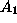. Later, an attempt is made to extend the previous analysis by searching with algorithm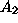. Unfortunately, the two algorithms have almost precisely the same inductive bias, making the second search redundant. Clear specifications of each algorithm's inductive bias could be used to prevent such redundancy.
• Oversearching: Recent results comparing induction algorithms employing heuristic search techniques with algorithms employing exhaustive search have shown that, paradoxically, algorithms using heuristic search produce models that are more accurate on new data. This phenomenon can be explained as an effect of multiple comparisons. Being able to account for the effects of multiple comparisons relies on being able to accurately characterize the search spaces of different algorithms.
These examples indicate why induced knowledge is more useful when linked to the inductive biases of available algorithms.

# Implications

Understanding statistical significance and inductive bias implies that knowledge management systems need to keep track of more than merely the final products of induction algorithms. Specifically, knowledge management systems should track:
• The size and identity of data samples used to induce particular models. That is, data management and knowledge management need to be linked.
• The number and types of models examined by induction algorithms. That is, induction algorithms and knowledge management need to be linked.
Certainly are special cases where these issues are of little concern. For example, if nearly unlimited data are available (e.g., the domain includes a simulation of low computational cost that can generate data on demand), then there is little reason to retain data after it has been used once, and models can always be verified based on new data. Similarly, if induced models are used once and then discarded (e.g., in domains where relationships change hourly or daily), then there is little need for long-term management of induced knowledge.

In many situations, however, long-term management of induced knowledge is desirable. We wish to build on previously induced relationships and make use of data and computational resources in the most efficient way possible. How can knowledge management systems provide the information needed to do this, without requiring knowledge management to be deeply integrated with other systems?

One way is to divide functions into components for knowledge management, data management, induction, and performance:
• The knowledge management component stores, organizes, and facilitates maintenance of represented knowledge. Each model contains a record, interpretable by the data management component, of the data sample used to induce it and a record, interpretable by the induction component, of the bias used to induce it.
• The data management component stores data used by the induction component, and provides records of the samples used to induce particular models.
• The induction component creates new models and provides records of the inductive bias used to induce them.
• The performance component makes inferences based on models in the knowledge management component.
Records of data samples are relatively simple to create. Each instance (e.g., a patient record in a medical database) can be assigned a unique integer, allowing a data sample to be characterized by a single vector of integers or a bitvector that partitions a unique sorting of a database into two groups. In other cases, where only some of the available variables are provided in a sample, a record of a sample might need to contain both a vector recording which instances were used and a vector recording which variables were used. Finally, if a pseudo-random sample of instances needs to be indicated, then recording the random seed and the total number of records in the sample would suffice to recreate the sample on demand.

Records of inductive bias are somewhat more problematic. Part of the inductive bias concerns representation language -- a constant for any individual induction algorithm. However, a compact record of the path of a heuristic search is not so simple to achieve. At a minimum, induction algorithms could record the raw number of models examined during search and rough limits of the search (e.g., the depth of a decision tree or the number of separate rules in an induced ruleset). Some interactive approaches to induction (e.g., visualization) have an inductive bias that is almost impossible to characterize. Even in these cases, however, records could be kept about the number and types of relationships explored.

Clearly, this discussion only sketches how a knowledge management system might be designed to accommodate inductive knowledge. However, it identifies some key characteristics of such a system -- links to both the induction and data management systems. Given the implications of statistical significance and inductive bias, these characteristics would seem essential to a system that effectively manages inductive knowledge.

# Acknowledgements

This research is supported by DARPA/Rome Laboratory under contract No. F30602-93-C-0076. The U.S. Government is authorized to reproduce and distribute reprints for governmental purposes notwithstanding any copyright notation hereon. The views and conclusions contained herein are those of the authors and should not be interpreted as necessarily representing the official policies or endorsements either expressed or implied, of the Defense Advanced Research Projects Agency, Rome Laboratory or the U.S. Government.

# About this document ...

This document was generated using the LaTeX2HTML translator Version 96.1 (Feb 5, 1996) Copyright © 1993, 1994, 1995, 1996, Nikos Drakos, Computer Based Learning Unit, University of Leeds.

© David Jensen